# Radiation View Factors (both analytical solutions and Nusselt Unit Sphere Method)

UPDATED: 08/13/2022

The first four worksheets are implementations of exactly the same analytical solutions as the view factor charts normally printed in textbooks (1, 2).   But users of those charts can rarely get better than two-digit accuracy.   View factor algebra often involves finding the difference of two very imprecise numbers.   These functions return values having an accuracy of four or five digits.   In addition, these four worksheets automatically draw a scaled schematic of the configuration to facilitate checking of the input.  Values found using the applications described here may be used in black body or diffuse-gray body calculations using the radiosity methodology implemented here.

Radiation View Factors – Perpendicular Plates

The first worksheet computes the view factor for perpendicular plates with a common edge (from the well-known analytical solution) and draws an isometric view of them on the screen. The user inputs the length of the common edge as well as the second dimension of the two plates.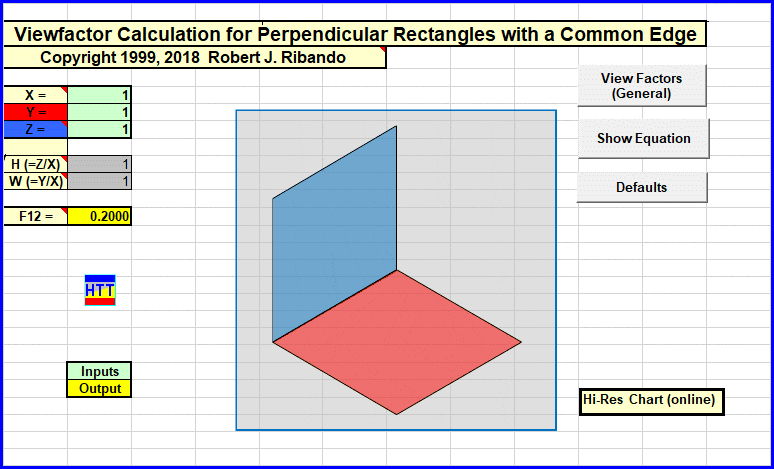Radiation View Factor Calculation for Perpendicular Rectangles with a Common Edge. Dimensions are input at the top left (light green boxes), that input geometry is drawn to scale in the center box and the view factor computed from the analytical solution for this geometry is returned in the yellow cell.

Radiation View Factors – Coaxial Disks

The view factor for coaxial, parallel disks is computed from the analytical solution and then they are drawn to scale on the screen. The user inputs the radii of the two disks (emitter and receiver) and the separation distance.   VBA coding provides the resulting value and a scaled drawing of the user input.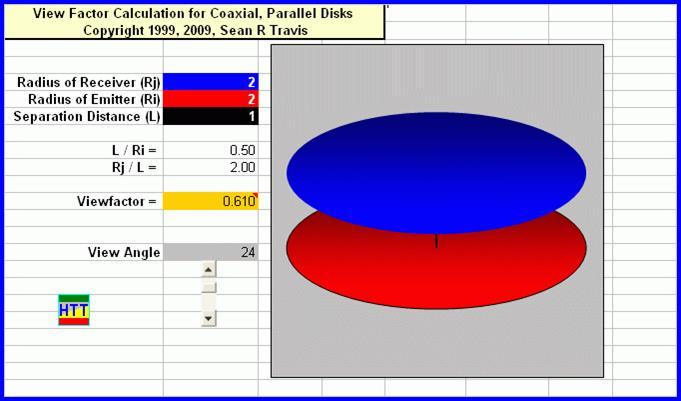Radiation View Factor Calculation for Coaxial, Parallel Disks. Dimensions and separation distance are input at the top left, that input geometry is drawn to scale in the center box and the view factor computed from the analytical solution for this geometry is returned in the yellow cell.

Radiation View Factors – Coaxial Cylinders

View factors for finite-length, coaxial cylinders are computed using a combination of analytical solutions and view factor algebra. The user inputs the radii of the two cylinders and their length. All nine relevant view factors and a scale drawing are returned.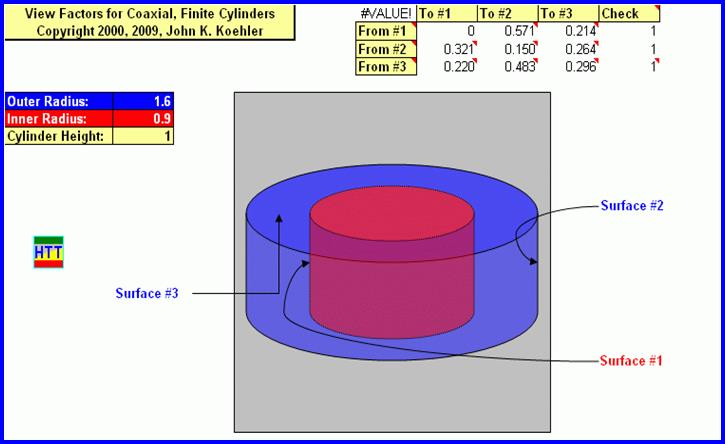Radiation View Factor Calculation for Coaxial, Finite Cylinders. Dimensions are input at the top left, that input geometry is drawn to scale in the center box and the view factors computed from the analytical solution for this geometry (a total of 9) are returned in the upper right.

Radiation View Factors – Parallel Rectangles

On the fourth worksheet the view factor for parallel, aligned plates is computed from the analytical solution. The user inputs the length and width of the two rectangles and their separation distance. The view factor and a scale drawing are returned.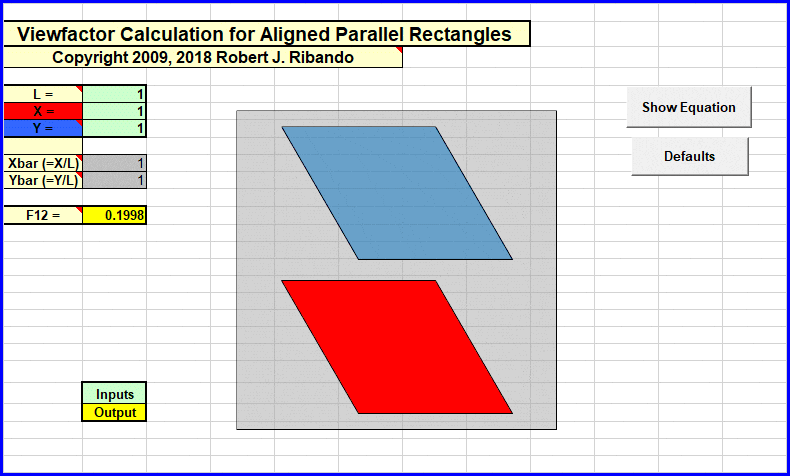Radiation View Factor Calculation for Aligned Parallel Rectangles. Dimensions are input at the top left (light green boxes), that input geometry is drawn to scale in the center box and the view factor computed from the analytical solution for this geometry is returned in the yellow cell.

Radiation View Factors – Nusselt Unit Sphere Method for Arbitrarily-Oriented Rectangles

The Nusselt Unit Sphere method was developed by Wilhelm Nusselt (for whom the Nusselt number is named) as an experimental method. His technique was converted to a computational algorithm by a NASA contractor. See below.

The implementation included in the View Factor Spreadsheet is limited to two arbitrarily oriented rectangles but could be extended to other geometries. The user enters coordinates defining the parallelograms and the algorithm returns the view factor. A plot of the input geometry allows the user to check their inputs.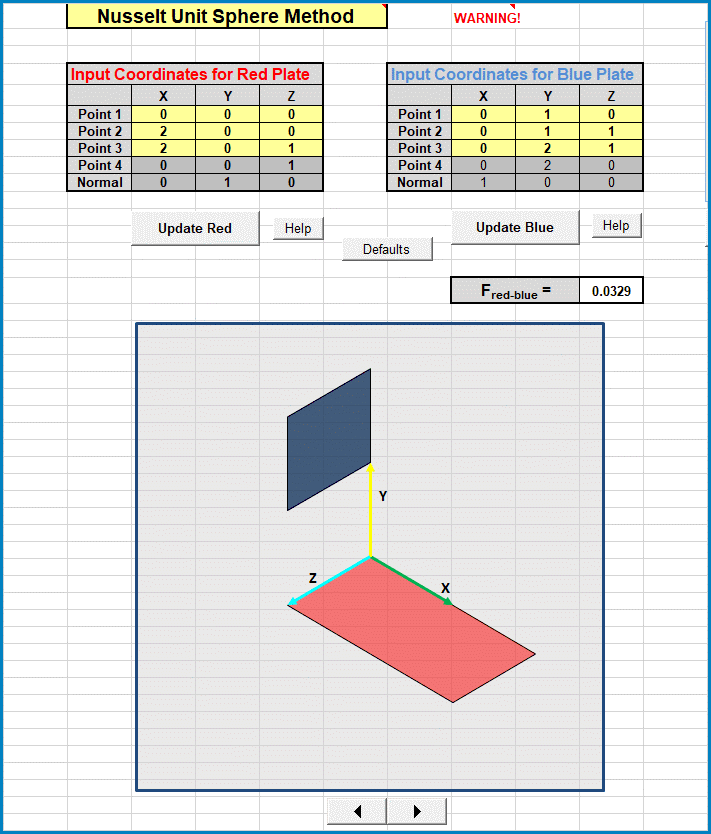Interface for Nusselt Unit Sphere Calculation of Radiation View Factors. The coordinates of the two parallelograms are input in the ranges at the top. The input geometry is plotted to scale in the window and the computed value of the view factor (from the red surface to the blue) is reported just above the right center.

Other Arrangements involving Rectangles

A separate workbook for rectangles in parallel planes and rectangles in perpendicular planes is also available: